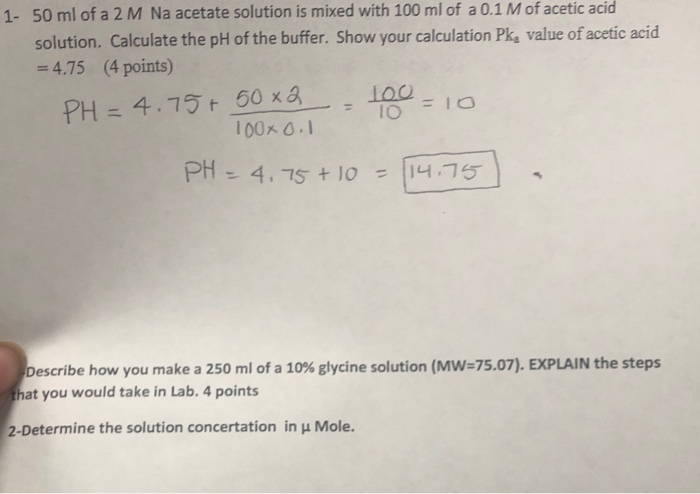# What quantity of 75 per cent acid solution must be mixed with a 25 solution to produce 480 mL of a 50 per cent solution

What quantity of 75 per cent acid solution must be mixed with a 25 solution to produce 480 mL of a 50 per cent solution?

.75x+.25(480-x)=480*.50 .75x+120-.25x=240 .5x=120 x=240 240 ml of each
##### Add Answer of: What quantity of 75 per cent acid solution must be mixed with a 25 solution to produce 480 mL of a 50 per cent solution
Similar Homework Help Questions
• ### what quantity x of a 65% acid solution must be mixed with a 20% solution to produce 300 mL of a 45% solution

what quantity x of a 65% acid solution must be mixed with a 20% solution to produce 300 mL of a 45% solution

• ### How many milliliters of a 75% acid solution must be added to 90 ml of a 10% acid solution to make a 25% acid solution

How many milliliters of a 75% acid solution must be added to 90 ml of a 10% acid solution to make a 25% acid solution?

• ### 60 ml of solution A is mixed with 120 ml of solution B to produce solution C which contains 8% of pure acid

60 ml of solution A is mixed with 120 ml of solution B to produce solution C which contains 8% of pure acid. If 80ml of solution A is mixed with 40ml of solution B, a solution D containing 10% of pure acid can be produced. find the percentage of pure acid in solution A?

• ### a chemist has one solution that is 25% acid and 50% acid

a chemist has one solution that is 25% acid and 50% acid. how many liters of each should be mixed to get 10 Liters of a solution that has 40% acid?

• ### how much 20% antifreeze solution must be mixed with a 40% antifreeze solution to produce 25 gallons of a 32% disinfectant solution

how much 20% antifreeze solution must be mixed with a 40% antifreeze solution to produce 25 gallons of a 32% disinfectant solution? round to the nearest gallon. ? gallons 20% solution, ? gallons 40% solution

• ### A chemist has a 25% and a 50% acid solution

A chemist has a 25% and a 50% acid solution. How much of each solution should be used to form 200 mL of a 35% acid solution?

• ### what mass of formic acid must be dissolved in 250 ml of deionized water to produce a solution of pH 1.83

what mass of formic acid must be dissolved in 250 ml of deionized water to produce a solution of pH 1.83?[ka of HCOOH=1.77*10^-4, Mw=46]

• ### If 7.74 mL a 0.154 M solution of oxalic acid is mixed with 7.15 mL of...

If 7.74 mL a 0.154 M solution of oxalic acid is mixed with 7.15 mL of water 6.12 mL of potassium permanganate, what is the new molarity of the oxalic acid--before a reaction takes place?

• ### precal

what quantity of a 60% acid solution must be mixed with a 30%solution to produce 300 mL of a 50% solution?

• ### 1- 50 ml of a 2 M Na acetate solution is mixed with 100 ml of...1- 50 ml of a 2 M Na acetate solution is mixed with 100 ml of a 0.1 M of acetic acid solution. Calculate the pH of the buffer. Show your calculation Pk, value of acetic acid = 4.75 (4 points) PH=4.75+ 50x2 - 100 = 10 100001 PH = 4.7 + 10 = 114.75 Describe how you make a 250 ml of a 10% glycine solution (MW=75.07). EXPLAIN the steps that you would take in Lab. 4 points 2-Determine...

Need Online Homework Help?